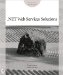# Passing an Array of Values to a Web Service

In addition to supporting parameters of various types (such as integer, double, and string), web service methods also support array parameters. The following SimpleStatistics web service supports three methods, each of which works with arrays of type double:

`double Sum(double Values()) double Average(double Values()) double Median(double Values())`

To create the SimpleStatistics web service, perform these steps:

1. Within Visual Studio .NET, select the File menu New Project option. Visual Studio .NET will display the New Project dialog box.

2. Within the New Project dialog box Project Types list, click Visual Basic Projects. Then, within the Templates field, click ASP.NET Web Service. Finally, within the Location field, specify the folder within which you want to store the program and the program name SimpleStatistics. Select OK. Visual Studio .NET will display a page onto which you can drag and drop the service’s components.

3. Select the View menu Code option. Visual Studio .NET will display the program’s source code. Within the source code add the following program statements:

`    <WebMethod()> Public Function Sum(ByVal Values As Double()) _ Ä   As Double         Dim Total As Double = 0.0         Dim Entry As Double         For Each Entry In Values             Total += Entry         Next         Sum = Total     End Function     <WebMethod()> Public Function Average(ByVal Values As Double()) _ Ä   As Double         Dim Total As Double = 0.0         Dim Entry As Double         For Each Entry In Values             Total += Entry         Next         Average = Total / (Values.Length)     End Function     <WebMethod()> Public Function Median(ByVal Values As Double()) _ Ä   As Double         Values.Sort(Values)         Median = Values(Values.Length / 2)     End Function`

4. After you enter the code, select the Build menu Build Solution option to create the service.

The Sum and Average methods are pretty straightforward. The Median method returns the value that appears in the middle of the array after the values have been sorted. For example, given the array of values 1, 2, 3, 4, 5, 6, 7, the median (middle) value is 4. Note that with an even number of elements, 1, 2, 3, 4, 5, 6, this implementation returns the number 4. Other implementations might return the value 3.

### Putting the SimpleStatistics Web Service to Use

The following Visual Basic .NET program, UseStats.vb, passes several different arrays to the SimpleStatistics web service. The program displays the array values along with the corresponding results, as shown in Figure 2.10.Figure 2.10: Calculating statistics using a web service that supports arrays

To create the UseStats.vb program, perform these steps:

1. Within Visual Studio .NET, select the File menu New Project option. Visual Studio .NET will display the New Project dialog box.

2. Within the New Project dialog box Project Types list, click Visual Basic Projects. Then, within the Templates field, click Windows Application. Within the Name and Location fields, type UseStats. Select OK. Visual Studio .NET will display a form onto which you can drag and drop the program’s controls (label, buttons, and text box).

3. Using the Toolbox, drag and drop the labels and text boxes previously shown in Figure 2.10 onto the page.

4. Select the Project menu Add Web Reference option. Visual Studio .NET will display the Add Web Reference dialog box.

5. Within the Address field, type localhost/SimpleStatistics/Service1.asmx?WSDL and press Enter. The dialog box will load the file’s contents. Click the Add Reference button.

6. Select the View menu Code option. Visual Studio .NET will display the program’s source code. Within the source code add the following program statements:

`    Private Sub Form1_Load(ByVal sender As System.Object, _ Ä  ByVal e As System.EventArgs) Handles MyBase.Load         Dim Values() As Double = {1.1, 5.5, 2.2, 4.4, 3.3, 6.6, 0.0}         Dim Entry As Double         Dim Sum, Average, Median As Double         Dim StatObject As New localhost.Service1()         For Each Entry In Values             TextBox1.Text &= Entry & vbCrLf         Next         Try             Sum = StatObject.Sum(Values)             Average = StatObject.Average(Values)             Median = StatObject.Median(Values)             TextBox2.Text = Sum.ToString()             TextBox3.Text = Average.ToString()             TextBox4.Text = Median.ToString()         Catch Ex As Exception             MessageBox.Show("Exception: " & Ex.Message)         End Try End Sub`

When the program first runs, the Form1_Load subroutine creates an array of values and an object the program will use to interact with the web service. The code first uses a For Each loop to display the array values within a text box. Then, the code calls the web service methods to calculate the “simple statistics.” The program displays each method’s result within a text box..NET Web Services Solutions
ISBN: 0782141722
EAN: 2147483647
Year: 2005
Pages: 161
Authors: Kris Jamsa